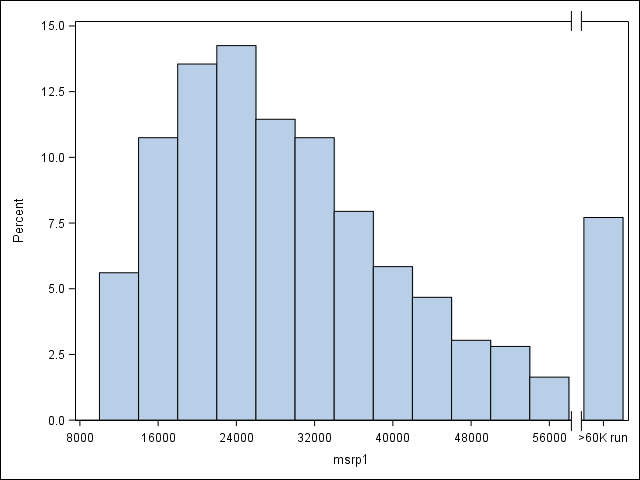## group extreme values in a single bin in a histogram

Hi,

I'm trying to create a histogram, and I want to group extreme values.

I created a format that looks like this :

``````proc format;
value hist_fmt  2000<-high = ">2000"
run;``````

I created a histogram with enterprise guide histogram wizard and tried also with sgplot.

``````proc sgplot data=dataset;
histogram var1;
format var1 hist_fmt.;
run;``````

the result that I had is repeated ">2000" value instead of grouping these values.

Any ideas to get the result ?

thanks !

1 ACCEPTED SOLUTION

Accepted Solutions

## Re: group extreme values in a single bin in a histogram

I think the GCHART procedure (requires SAS/GRAPH) allows for more flexibility regarding the bins of a histogram.

Example:

``````data dataset;
do _n_=1 to 200;
var1=2500*ranuni(31416);
output;
end;
run;

proc gchart data=dataset;
format var1 hist_fmt.;
vbar var1 / midpoints=100 to 2100 by 200;
run;
quit;``````

Even without the FORMAT statement the resulting histogram would group the "extreme" values (>=2000) into the rightmost bin, but the label of that bin would then be displayed as "2100" rather than ">2000".

Please note that in the above example the exact value 2000 would be assigned to the ">2000" category. If this was an issue, you could extend your format definition to cover the whole range of possible VAR1 values and then use the DISCRETE option of the VBAR statement. Thus, the bins would correspond 1:1 to the format categories. In particular, only values >2000 would go into the ">2000" bin.

Example:

``````proc format;
value hist_fmt
0<-  500 = '   0<- 500'
500<- 1000 = ' 500<-1000'
1000<- 1500 = '1000<-1500'
1500<- 2000 = '1500<-2000'
2000<- high = '>2000'
;
run;

proc gchart data=dataset;
format var1 hist_fmt.;
vbar var1 / discrete;
run;
quit;``````

However, with the extended format you could also use PROC SGPLOT:

``````proc sgplot data=dataset;
format var1 hist_fmt.;
vbar var1;
run;
``````

4 REPLIES 4

## Re: group extreme values in a single bin in a histogram

I think the GCHART procedure (requires SAS/GRAPH) allows for more flexibility regarding the bins of a histogram.

Example:

``````data dataset;
do _n_=1 to 200;
var1=2500*ranuni(31416);
output;
end;
run;

proc gchart data=dataset;
format var1 hist_fmt.;
vbar var1 / midpoints=100 to 2100 by 200;
run;
quit;``````

Even without the FORMAT statement the resulting histogram would group the "extreme" values (>=2000) into the rightmost bin, but the label of that bin would then be displayed as "2100" rather than ">2000".

Please note that in the above example the exact value 2000 would be assigned to the ">2000" category. If this was an issue, you could extend your format definition to cover the whole range of possible VAR1 values and then use the DISCRETE option of the VBAR statement. Thus, the bins would correspond 1:1 to the format categories. In particular, only values >2000 would go into the ">2000" bin.

Example:

``````proc format;
value hist_fmt
0<-  500 = '   0<- 500'
500<- 1000 = ' 500<-1000'
1000<- 1500 = '1000<-1500'
1500<- 2000 = '1500<-2000'
2000<- high = '>2000'
;
run;

proc gchart data=dataset;
format var1 hist_fmt.;
vbar var1 / discrete;
run;
quit;``````

However, with the extended format you could also use PROC SGPLOT:

``````proc sgplot data=dataset;
format var1 hist_fmt.;
vbar var1;
run;
``````Jay54
Meteorite | Level 14

## Re: group extreme values in a single bin in a histogram

You can use the solution provided previously using VBAR.  But can we do this with the Histogram statement?  Yes, for a custom solution with very specific data.  Here the actual values > 58000 are changed, and we have used the format and the axis break. Note axis break symbol on both X & X2 axes in the attached graph.

proc format;
value hist_fmt 60000<-high = ">60K"
run;

data cars;
set sashelp.cars;
msrp1=msrp;
if msrp>58000 then msrp1=64000;
run;

proc sgplot data=cars;
styleattrs axisbreak=bracket;
histogram msrp1 / binstart=8000 binwidth=4000;
format msrp1 hist_fmt.;
xaxis values=(8000 to 64000 by 4000) ranges=(8000-58000 62000-66000);
run;## Re: group extreme values in a single bin in a histogram

@Jay54

In fact I'am new to SAS Graphics, and I found it strange that a histogram task in enterprise guide is in fact a barplot, that's why I prefer Sgplot. However It would be good if activex can be activated with sgplot. I don't know if it can be done.

MK

## Re: group extreme values in a single bin in a histogram

Thanks @FreelanceReinh for the answer. Midpoints works well, especially that it can be added from the histogram task in enterprise miner.

MK

Discussion stats
• 4 replies
• 2178 views
• 3 likes
• 3 in conversation